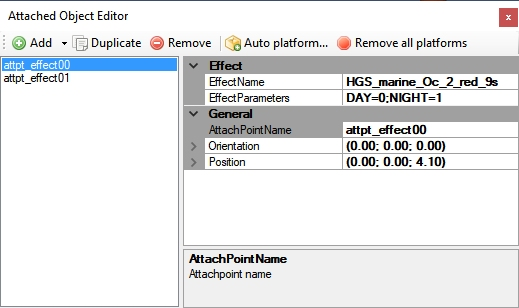# How do you make an effetc NOT show at daytime?

#### Thralni

I'm making a floodlight and have successfully added a dynamic spotlight as well as a, an orb-like glow effect. However, although spotlight is inactive during daytime, I cna't seme to figure out how the glow effect cna be swicthed off during daytime. It seems like the easiest, most straightforward thing, yet i don't see anything pertaining to day and night. I'm working off other effects, some default, others third party, and I *think* I'm doing it right - but apparently not. Here's the code for the effect:

Code:
``````[Library Effect]
Version=2.00
Display Name=fnlu_flood_y
Priority=0

[Properties]

[Emitter.0]
Delay=0.00, 0.00
Bounce=0.00
Rate=1.00, 1.00
X Emitter Velocity=0.00, 0.00
Y Emitter Velocity=0.00, 0.00
Z Emitter Velocity=0.00, 0.00
Drag=0.00, 0.00
X Particle Velocity=0.00, 0.00
Y Particle Velocity=0.00, 0.00
Z Particle Velocity=0.00, 0.00
X Rotation=0.00, 0.00
Y Rotation=0.00, 0.00
Z Rotation=0.00, 0.00
X Offset=0.00, 0.00
Y Offset=0.00, 0.00
Z Offset=0.00, 0.00
Pitch=0.00, 0.00
Bank=0.00, 0.00

[Particle.0]
Type=19
X Scale=2.50, 2.50
Y Scale=2.50, 2.50
Z Scale=0.00, 0.00
X Scale Rate=0.00, 0.00
Y Scale Rate=0.00, 0.00
Z Scale Rate=0.00, 0.00
Drag=0.00, 0.00
Color Rate=0.00, 0.00
X Offset=0.00, 0.00
Y Offset=0.00, 0.00
Z Offset=0.00, 0.00
Rotation=0.00, 0.00
Static=1
Face=1, 0, 1

[ParticleAttributes.0]
Blend Mode=2
Texture=Light_yellow.bmp
Bounce=0.00
Color Start=255, 236, 0, 120
Color End=255, 236, 0, 0
Jitter Distance=0.00
Jitter Time=0.00
uv1=0.00, 0.00
uv2=1.00, 1.00
X Scale Goal=0.00
Y Scale Goal=0.00
Z Scale Goal=0.00
Extrude Length=0.00
Extrude Pitch Max=0.00
Any ideas?

#### robystar

With an xml for placement of the effect where you add:
effectParams= The parameters of the effect. For example, the following effect will appear at midnight on January 1st every year and exist for 20 minutes:
For instance:
<Effect effectName="fx_ForestFire" effectParams="MOY=01,01;DOM=01,01;HOD=00,00;MOH=00,20;"/>

Then compile as a bgl, of course.

.

Last edited:

#### hgschnell

place the effect twice
DAWN=1;
DUSK=1;
and
NIGHT=1;
DAY=0;

#### Thralni

Aaah... Makes sense that I should be looking at the attach point... I assume that's what you mean by "placement xml", correct?

Currently I have a controller activating both a dynamic spotlight and the halo effect. So, I should separate the halo effect from the controller, make a NEW attach point with the timing parameters, and attach the halo effect to that?

#### hgschnell

read the SDK Special Effects for your FS version (FS9, FSX or what ever...)
the controller effect shoud have the effect params.#### Thralni

Agh! I was looking over the relevant SDK files so closely that I must have gotten blind. That was very easy, thank you for your help Guenther! Apologies fort asking what amounted to a rather inane question :/